GFG App
Open AppBrowser
Continue

# Longest Common Subsequence (LCS)

Given two strings, S1 and S2, the task is to find the length of the longest subsequence present in both of the strings.

Note: A subsequence of a string is a sequence that is generated by deleting some characters (possibly 0) from the string without altering the order of the remaining characters. For example, “abc”, “abg”, “bdf”, “aeg”, ‘”acefg”, etc are subsequences of the string “abcdefg”.

Examples:

Input: S1 = “AGGTAB”, S2 = “GXTXAYB”
Output: 4
Explanation: The longest subsequence which is present in both strings is “GTAB”.

Input: S1 = “ABCDGH”, S2 = “AEDFHR”
Output: 3
Explanation: The longest subsequence which is present in both strings is “ADH”.

Recommended Practice

## Naive Approach for LCS:

The problem can be solved using recursion based on the following idea:

Generate all the possible subsequences and find the longest among them that is present in both strings.

Below is the Implementation of the Approach

## C

 `#include ` `#include `   `int` `max(``int` `a, ``int` `b) { ``return` `(a > b) ? a : b; }`   `int` `lcs(``char``* X, ``char``* Y, ``int` `m, ``int` `n)` `{` `    ``if` `(m == 0 || n == 0)` `        ``return` `0;` `    ``if` `(X[m - 1] == Y[n - 1])` `        ``return` `1 + lcs(X, Y, m - 1, n - 1);` `    ``else` `        ``return` `max(lcs(X, Y, m, n - 1),` `                   ``lcs(X, Y, m - 1, n));` `}`   `int` `main()` `{` `    ``char` `X[] = ``"AGGTAB"``;` `    ``char` `Y[] = ``"GXTXAYB"``;`   `    ``int` `m = ``strlen``(X);` `    ``int` `n = ``strlen``(Y);`   `    ``int` `length = lcs(X, Y, m, n);` `    ``printf``(``"Length of LCS: %d\n"``, length);`   `    ``return` `0;` `}`

## Java

 `/*package whatever //do not write package name here */`   `import` `java.io.*;` `import` `java.util.*;`   `public` `class` `GFG {`   `    ``public` `static` `void` `main(String[] args)` `    ``{` `        ``String str1 = ``"AGGTAB"``;` `        ``String str2 = ``"GXTXAYB"``;` `        ``String lcs = longestCommonSubsequence(str1, str2);` `        ``System.out.println(``"Length of LCS is "` `                           ``+ lcs.length());` `    ``}`   `    ``public` `static` `String` `    ``longestCommonSubsequence(String str1, String str2)` `    ``{` `        ``List subsequences1` `            ``= generateSubsequences(str1);` `        ``List subsequences2` `            ``= generateSubsequences(str2);`   `        ``String lcs = ``""``;` `        ``for` `(String subsequence1 : subsequences1) {` `            ``for` `(String subsequence2 : subsequences2) {` `                ``if` `(subsequence1.equals(subsequence2)` `                    ``&& subsequence1.length()` `                           ``> lcs.length()) {` `                    ``lcs = subsequence1;` `                ``}` `            ``}` `        ``}` `        ``return` `lcs;` `    ``}`   `    ``public` `static` `List` `    ``generateSubsequences(String str)` `    ``{` `        ``List subsequences = ``new` `ArrayList<>();` `        ``generateSubsequencesHelper(str, ``""``, ``0``,` `                                   ``subsequences);` `        ``return` `subsequences;` `    ``}`   `    ``public` `static` `void` `generateSubsequencesHelper(` `        ``String str, String subsequence, ``int` `index,` `        ``List subsequences)` `    ``{` `        ``if` `(index == str.length()) {` `            ``subsequences.add(subsequence);` `            ``return``;` `        ``}` `        ``generateSubsequencesHelper(str, subsequence,` `                                   ``index + ``1``, subsequences);` `        ``generateSubsequencesHelper(` `            ``str, subsequence + str.charAt(index), index + ``1``,` `            ``subsequences);` `    ``}` `}`

## Python3

 `from` `typing ``import` `List`   `def` `longest_common_subsequence(str1: ``str``, str2: ``str``) ``-``> ``str``:` `    ``subsequences1 ``=` `generate_subsequences(str1)` `    ``subsequences2 ``=` `generate_subsequences(str2)` `    ``lcs ``=` `""` `    ``for` `subsequence1 ``in` `subsequences1:` `        ``for` `subsequence2 ``in` `subsequences2:` `            ``if` `subsequence1 ``=``=` `subsequence2 ``and` `len``(subsequence1) > ``len``(lcs):` `                ``lcs ``=` `subsequence1` `    ``return` `lcs`   `def` `generate_subsequences(s: ``str``) ``-``> ``List``[``str``]:` `    ``subsequences ``=` `[]` `    ``generate_subsequences_helper(s, "", ``0``, subsequences)` `    ``return` `subsequences`   `def` `generate_subsequences_helper(s: ``str``, subsequence: ``str``, index: ``int``, subsequences: ``List``[``str``]) ``-``> ``None``:` `    ``if` `index ``=``=` `len``(s):` `        ``subsequences.append(subsequence)` `        ``return` `    ``generate_subsequences_helper(s, subsequence, index ``+` `1``, subsequences)` `    ``generate_subsequences_helper(s, subsequence ``+` `s[index], index ``+` `1``, subsequences)`   `if` `__name__ ``=``=` `'__main__'``:` `    ``str1 ``=` `"AGGTAB"` `    ``str2 ``=` `"GXTXAYB"` `    ``lcs ``=` `longest_common_subsequence(str1, str2)` `    ``print``(``"Length of LCS is "``, ``len``(lcs))`

## C#

 `using` `System;` `using` `System.Collections.Generic;`   `public` `class` `GFG {` `    ``public` `static` `void` `Main(``string``[] args)` `    ``{` `        ``string` `str1 = ``"AGGTAB"``;` `        ``string` `str2 = ``"GXTXAYB"``;` `        ``string` `lcs = LongestCommonSubsequence(str1, str2);` `        ``Console.WriteLine(``"Length of LCS is "` `+ lcs.Length);` `    ``}` `    ``public` `static` `string` `    ``LongestCommonSubsequence(``string` `str1, ``string` `str2)` `    ``{` `        ``List<``string``> subsequences1` `            ``= GenerateSubsequences(str1);` `        ``List<``string``> subsequences2` `            ``= GenerateSubsequences(str2);`   `        ``string` `lcs = ``""``;` `        ``foreach``(``string` `subsequence1 ``in` `subsequences1)` `        ``{` `            ``foreach``(``string` `subsequence2 ``in` `subsequences2)` `            ``{` `                ``if` `(subsequence1.Equals(subsequence2)` `                    ``&& subsequence1.Length > lcs.Length) {` `                    ``lcs = subsequence1;` `                ``}` `            ``}` `        ``}` `        ``return` `lcs;` `    ``}`   `    ``public` `static` `List<``string``>` `    ``GenerateSubsequences(``string` `str)` `    ``{` `        ``List<``string``> subsequences = ``new` `List<``string``>();` `        ``GenerateSubsequencesHelper(str, ``""``, 0,` `                                   ``subsequences);` `        ``return` `subsequences;` `    ``}`   `    ``public` `static` `void` `GenerateSubsequencesHelper(` `        ``string` `str, ``string` `subsequence, ``int` `index,` `        ``List<``string``> subsequences)` `    ``{` `        ``if` `(index == str.Length) {` `            ``subsequences.Add(subsequence);` `            ``return``;` `        ``}` `        ``GenerateSubsequencesHelper(str, subsequence,` `                                   ``index + 1, subsequences);` `        ``GenerateSubsequencesHelper(str,` `                                   ``subsequence + str[index],` `                                   ``index + 1, subsequences);` `    ``}` `}` `// This code is contributed by user_dtewbxkn77n`

Output

`Length of LCS is 4`

Time Complexity: O(n * 2n)

• Let us count the total subsequences with lengths 1, 2, . . . , n-1, n.
• From theory of permutation and combination we know number of combinations with 1 element is nC1. Number of combinations with 2 elements are nC2 and so on.
• So a string of length n has nC1 + nC2 + . . . nCn = 2n-1 different possible subsequences.
• Each subsequence takes O(n) time to compare.

Auxiliary Space: O(n) As we can reuse the same string.

## Recursive Approach for LCS:

We can further improve the solution of LCS by utilizing the following observation:

Let the input sequences be X[0 . . . m-1] and Y[0 . . . n-1] of lengths m and n respectively. And let L(X[0 . . . m-1], Y[0 . . . n-1]) be the length of the LCS of the two strings X and Y.

Following is the recursive definition of L(X[0 . . . m-1], Y[0 . . . n-1]).

• If the last characters of both sequences match (or X[m-1] = Y[n-1]) then
L(X[0 . . . m-1], Y[0 . . . n-1]) = 1 + L(X[0 . . . m-2], Y[0 . . . n-2])
• If last characters of both sequences do not match then
L(X[0 . . . m-1], Y[0 . . . n-1]) = MAX ( L(X[0 . . . m-2], Y[0 . . . n-1]), L(X[0 . . . m-1], Y[0 . . . n-2]) )

Follow the below steps to implement the idea:

• Create a recursive function [say lcs()].
• Check the relation between the last characters of the strings that are not yet processed.
• Depending on the relation call the next recursive function as mentioned above.

Below is the implementation of the recursive approach:

## C

 `// A Naive recursive implementation of LCS problem`   `#include `   `int` `max(``int` `a, ``int` `b);`   `// Returns length of LCS for X[0..m-1], Y[0..n-1]` `int` `lcs(``char``* X, ``char``* Y, ``int` `m, ``int` `n)` `{` `    ``if` `(m == 0 || n == 0)` `        ``return` `0;` `    ``if` `(X[m - 1] == Y[n - 1])` `        ``return` `1 + lcs(X, Y, m - 1, n - 1);` `    ``else` `        ``return` `max(lcs(X, Y, m, n - 1),` `                   ``lcs(X, Y, m - 1, n));` `}`   `// Utility function to get max of 2 integers` `int` `max(``int` `a, ``int` `b) { ``return` `(a > b) ? a : b; }`   `// Driver code` `int` `main()` `{` `    ``char` `S1[] = ``"AGGTAB"``;` `    ``char` `S2[] = ``"GXTXAYB"``;` `    ``int` `m = ``strlen``(S1);` `    ``int` `n = ``strlen``(S2);` `  `  `    ``printf``(``"Length of LCS is %d"``, lcs(S1, S2, m, n));`   `    ``return` `0;` `}`

## C++

 `// A Naive recursive implementation of LCS problem`   `#include ` `using` `namespace` `std;`   `// Returns length of LCS for X[0..m-1], Y[0..n-1]` `int` `lcs(string X, string Y, ``int` `m, ``int` `n)` `{` `    ``if` `(m == 0 || n == 0)` `        ``return` `0;` `    ``if` `(X[m - 1] == Y[n - 1])` `        ``return` `1 + lcs(X, Y, m - 1, n - 1);` `    ``else` `        ``return` `max(lcs(X, Y, m, n - 1),` `                   ``lcs(X, Y, m - 1, n));` `}`   `// Driver code` `int` `main()` `{` `    ``string S1 = ``"AGGTAB"``;` `    ``string S2 = ``"GXTXAYB"``;` `    ``int` `m = S1.size();` `    ``int` `n = S2.size();`   `    ``cout << ``"Length of LCS is "` `<< lcs(S1, S2, m, n);`   `    ``return` `0;` `}`   `// This code is contributed by rathbhupendra`

## Java

 `// A Naive recursive implementation of LCS problem in java`   `import` `java.io.*;` `import` `java.util.*;`   `public` `class` `LongestCommonSubsequence {`   `    ``// Returns length of LCS for X[0..m-1], Y[0..n-1]` `    ``int` `lcs(String X, String Y, ``int` `m, ``int` `n)` `    ``{` `        ``if` `(m == ``0` `|| n == ``0``)` `            ``return` `0``;` `        ``if` `(X.charAt(m - ``1``) == Y.charAt(n - ``1``))` `            ``return` `1` `+ lcs(X, Y, m - ``1``, n - ``1``);` `        ``else` `            ``return` `max(lcs(X, Y, m, n - ``1``),` `                       ``lcs(X, Y, m - ``1``, n));` `    ``}`   `    ``// Utility function to get max of 2 integers` `    ``int` `max(``int` `a, ``int` `b) { ` `        ``return` `(a > b) ? a : b; ` `    ``}`   `    ``// Driver code` `    ``public` `static` `void` `main(String[] args)` `    ``{` `        ``LongestCommonSubsequence lcs` `            ``= ``new` `LongestCommonSubsequence();` `        ``String S1 = ``"AGGTAB"``;` `        ``String S2 = ``"GXTXAYB"``;` `        ``int` `m = S1.length();` `        ``int` `n = S2.length();`   `        ``System.out.println(``"Length of LCS is"` `                           ``+ ``" "` `+ lcs.lcs(S1, S2, m, n));` `    ``}` `}`   `// This Code is Contributed by Saket Kumar`

## Python3

 `# A Naive recursive Python implementation of LCS problem`     `def` `lcs(X, Y, m, n):` `    ``if` `m ``=``=` `0` `or` `n ``=``=` `0``:` `        ``return` `0` `    ``elif` `X[m``-``1``] ``=``=` `Y[n``-``1``]:` `        ``return` `1` `+` `lcs(X, Y, m``-``1``, n``-``1``)` `    ``else``:` `        ``return` `max``(lcs(X, Y, m, n``-``1``), lcs(X, Y, m``-``1``, n))`     `# Driver code` `if` `__name__ ``=``=` `'__main__'``:` `    ``S1 ``=` `"AGGTAB"` `    ``S2 ``=` `"GXTXAYB"` `    ``print``(``"Length of LCS is"``, lcs(S1, S2, ``len``(S1), ``len``(S2)))`

## C#

 `// C# Naive recursive implementation of LCS problem` `using` `System;`   `class` `GFG {` `  `  `    ``// Returns length of LCS for X[0..m-1], Y[0..n-1]` `    ``static` `int` `lcs(String X, String Y, ``int` `m, ``int` `n)` `    ``{` `        ``if` `(m == 0 || n == 0)` `            ``return` `0;` `        ``if` `(X[m - 1] == Y[n - 1])` `            ``return` `1 + lcs(X, Y, m - 1, n - 1);` `        ``else` `            ``return` `max(lcs(X, Y, m, n - 1),` `                       ``lcs(X, Y, m - 1, n));` `    ``}`   `    ``// Utility function to get max of 2 integers` `    ``static` `int` `max(``int` `a, ``int` `b) { ` `        ``return` `(a > b) ? a : b; ` `    ``}`   `    ``// Driver code` `    ``public` `static` `void` `Main()` `    ``{` `        ``String S1 = ``"AGGTAB"``;` `        ``String S2 = ``"GXTXAYB"``;` `        ``int` `m = S1.Length;` `        ``int` `n = S2.Length;`   `        ``Console.Write(``"Length of LCS is"` `                      ``+ ``" "` `+ lcs(S1, S2, m, n));` `    ``}` `}` `// This code is Contributed by Sam007`

## PHP

 ` `

## Javascript

 ``

Output

`Length of LCS is 4`

Time Complexity: O(2n)
Auxiliary Space: O(1)

## Memoization Approach for LCS:

If we notice carefully, we can observe that the above recursive solution holds the following two properties:

### Optimal Substructure:

See for solving the structure of L(X[0, 1, . . ., m-1], Y[0, 1, . . . , n-1]) we are taking the help of the substructures of X[0, 1, …, m-2], Y[0, 1,…, n-2], depending on the situation (i.e., using them optimally) to find the solution of the whole.

### Overlapping Subproblems:

If we use the above recursive approach for strings “AXYT” and “AYZX“, we will get a partial recursion tree as shown below. Here we can see that the subproblems L(“AXY”, “AYZ”) is being calculated more than once. If the total tree is considered there will be several such overlapping subproblems.

L(“AXYT”, “AYZX”)
/                                     \
L(“AXY”, “AYZX”)                         L(“AXYT”, “AYZ”)
/                            \                             /                      \
L(“AX”, “AYZX”) L(“AXY”, “AYZ”)   L(“AXY”, “AYZ”)  L(“AXYT”, “AY”)

Approach: Because of the presence of these two properties we can use Dynamic programming or Memoization to solve the problem. Below is the approach for solution using recursion.

Create a recursive function. Also create a 2D array to store the result of a unique state. During the recursion call, if the same state is called more than once, then we can directly return the answer stored for that state instead of calculating again.

Following is the memoization implementation for the LCS problem.

## C++

 `/* A Top-Down DP implementation of LCS problem */` `#include ` `using` `namespace` `std;`   `/* Returns length of LCS for X[0..m-1], Y[0..n-1] */` `int` `lcs(``char``* X, ``char``* Y, ``int` `m, ``int` `n,` `        ``vector >& dp)` `{` `    ``if` `(m == 0 || n == 0)` `        ``return` `0;` `    ``if` `(X[m - 1] == Y[n - 1])` `        ``return` `dp[m][n] = 1 + lcs(X, Y, m - 1, n - 1, dp);`   `    ``if` `(dp[m][n] != -1) {` `        ``return` `dp[m][n];` `    ``}` `    ``return` `dp[m][n] = max(lcs(X, Y, m, n - 1, dp),` `                          ``lcs(X, Y, m - 1, n, dp));` `}`   `/* Driver code */` `int` `main()` `{` `    ``char` `X[] = ``"AGGTAB"``;` `    ``char` `Y[] = ``"GXTXAYB"``;`   `    ``int` `m = ``strlen``(X);` `    ``int` `n = ``strlen``(Y);` `    ``vector > dp(m + 1, vector<``int``>(n + 1, -1));` `    ``cout << ``"Length of LCS is "` `<< lcs(X, Y, m, n, dp);`   `    ``return` `0;` `}`

## Java

 `/*package whatever //do not write package name here */` `import` `java.io.*;`   `class` `GFG` `{` `  `  `  ``// A Top-Down DP implementation of LCS problem`   `  ``// Returns length of LCS for X[0..m-1], Y[0..n-1]` `  ``static` `int` `lcs(String X,String Y,``int` `m,``int` `n,``int``[][] dp){`   `    ``if` `(m == ``0` `|| n == ``0``)` `      ``return` `0``;`   `    ``if` `(dp[m][n] != -``1``)` `      ``return` `dp[m][n];`   `    ``if``(X.charAt(m - ``1``) == Y.charAt(n - ``1``)){` `      ``dp[m][n] = ``1` `+ lcs(X, Y, m - ``1``, n - ``1``, dp);` `      ``return` `dp[m][n];` `    ``}`   `    ``dp[m][n] = Math.max(lcs(X, Y, m, n - ``1``, dp),lcs(X, Y, m - ``1``, n, dp));` `    ``return` `dp[m][n];` `  ``}`   `  ``// Drivers code` `  ``public` `static` `void` `main(String args[]){`   `    ``String X = ``"AGGTAB"``;` `    ``String Y = ``"GXTXAYB"``;`   `    ``int` `m = X.length();` `    ``int` `n = Y.length();` `    ``int``[][] dp = ``new` `int``[m + ``1``][n + ``1``];` `    ``for``(``int` `i=``0``;i

## Python3

 `# A Top-Down DP implementation of LCS problem`   `# Returns length of LCS for X[0..m-1], Y[0..n-1] ` `def` `lcs(X, Y, m, n, dp):`   `    ``if` `(m ``=``=` `0` `or` `n ``=``=` `0``):` `        ``return` `0`   `    ``if` `(dp[m][n] !``=` `-``1``):` `        ``return` `dp[m][n]`   `    ``if` `X[m ``-` `1``] ``=``=` `Y[n ``-` `1``]:` `        ``dp[m][n] ``=` `1` `+` `lcs(X, Y, m ``-` `1``, n ``-` `1``, dp)` `        ``return` `dp[m][n]`   `    ``dp[m][n] ``=` `max``(lcs(X, Y, m, n ``-` `1``, dp),lcs(X, Y, m ``-` `1``, n, dp))` `    ``return` `dp[m][n]`   `# Driver code`   `X ``=` `"AGGTAB"` `Y ``=` `"GXTXAYB"`   `m ``=` `len``(X)` `n ``=` `len``(Y)` `dp ``=` `[[``-``1` `for` `i ``in` `range``(n ``+` `1``)]``for` `j ``in` `range``(m ``+` `1``)]`   `print``(f``"Length of LCS is {lcs(X, Y, m, n, dp)}"``)`   `# This code is contributed by shinjanpatra`

## C#

 `/* C# Naive recursive implementation of LCS problem */` `using` `System;`   `class` `GFG {`     `    ``/* Returns length of LCS for X[0..m-1], Y[0..n-1] */` `    ``static` `int` `lcs(``char``[] X, ``char``[] Y, ``int` `m, ``int` `n, ``int` `[,]L) {` `        ``if` `(m == 0 || n == 0)` `            ``return` `0;`   `        ``if` `(L[m, n] != -1)` `            ``return` `L[m, n];`   `        ``if` `(X[m - 1] == Y[n - 1]) {` `            ``L[m, n] = 1 + lcs(X, Y, m - 1, n - 1, L);` `            ``return` `L[m, n];` `        ``}`   `        ``L[m, n] = max(lcs(X, Y, m, n - 1, L), lcs(X, Y, m - 1, n, L));` `        ``return` `L[m, n];` `    ``}`   `    ``/* Utility function to get max of 2 integers */` `    ``static` `int` `max(``int` `a, ``int` `b) {` `        ``return` `(a > b) ? a : b;` `    ``}`   `    ``public` `static` `void` `Main() {` `        ``String s1 = ``"AGGTAB"``;` `        ``String s2 = ``"GXTXAYB"``;`   `        ``char``[] X = s1.ToCharArray();` `        ``char``[] Y = s2.ToCharArray();` `        ``int` `m = X.Length;` `        ``int` `n = Y.Length;` `        ``int``[,]L = ``new` `int``[m + 1, n + 1];` `        ``for` `(``int` `i = 0; i <= m; i++)` `        ``{` `            ``for` `(``int` `j = 0; j <= n; j++)` `            ``{` `                ``L[i, j] = -1;` `            ``}` `        ``}` `        ``Console.Write(``"Length of LCS is"` `+ ``" "` `            ``+ lcs(X, Y, m, n, L));` `    ``}` `}`   `// This code is contributed by akshitsaxenaa09`

## Javascript

 ``

Output

`Length of LCS is 4`

Time Complexity: O(m * n) where m and n are the string lengths.
Auxiliary Space: O(m * n) here the recursive stack space is ignored.

## Dynamic Programming for LCS:

We can use the following steps to implement the dynamic programming approach for LCS.

• Create a 2D array dp[][] with rows and columns equal to the length of each input string plus 1 [the number of rows indicates the indices of S1 and the columns indicate the indices of S2].
• Initialize the first row and column of the dp array to 0.
• Iterate through the rows of the dp array, starting from 1 (say using iterator i).
• For each i, iterate all the columns from j = 1 to n:
• If S1[i-1] is equal to S2[j-1], set the current element of the dp array to the value of the element to (dp[i-1][j-1] + 1).
• Else, set the current element of the dp array to the maximum value of dp[i-1][j] and dp[i][j-1].
• After the nested loops, the last element of the dp array will contain the length of the LCS.

See the below illustration for a better understanding:

Illustration:

Say the strings are S1 = “AGGTAB” and S2 = “GXTXAYB”.

First step: Initially create a 2D matrix (say dp[][]) of  size 8 x 7 whose first row and first column are filled with 0.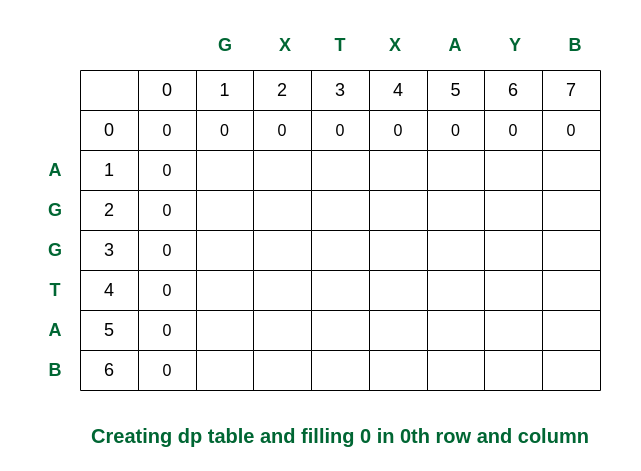Creating the dp table

Second step: Traverse for i = 1. When j becomes 5, S1 and S2 are equal. So the dp[][] is updated. For the other elements take the maximum of dp[i-1][j] and dp[i][j-1]. (In this case, if both values are equal, we have used arrows to the previous rows).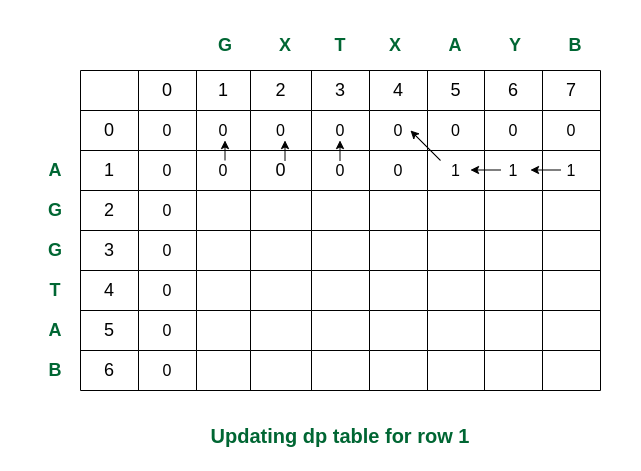Filling the row no 1

Third step: While traversed for i = 2, S1 and S2 are the same (both are ‘G’). So the dp value in that cell is updated. Rest of the elements are updated as per the conditions.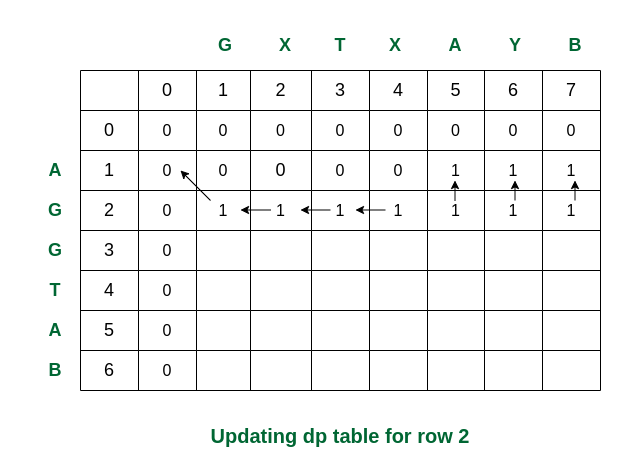Filling the row no. 2

Fourth step: For i = 3, S1 and S2 are again same. The updations are as follows.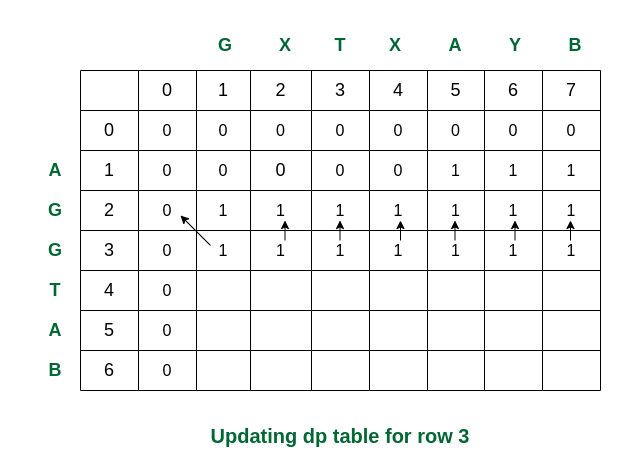Filling row no. 3

Fifth step: For i = 4, we can see that S1 and S2 are same. So dp updated as dp + 1 = 2.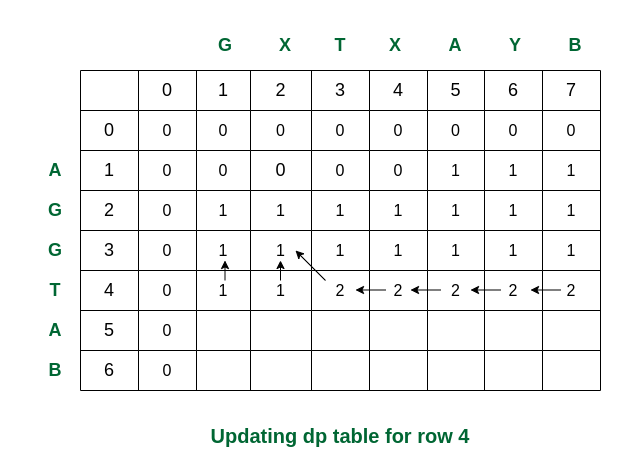Filling row 4

Sixth step: Here we can see that for i = 5 and j = 5 the values of S1 and S2 are same (i.e., both are ‘A’). So dp is updated accordingly and becomes 3.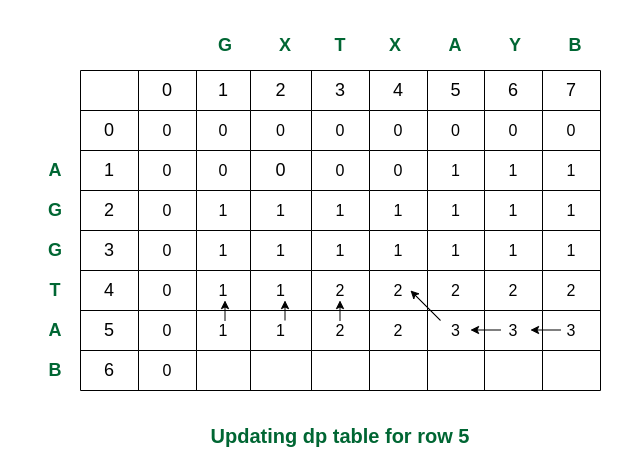Filling row 5

Final step: For i = 6, see the last characters of both strings are same (they are ‘B’). Therefore the value of dp becomes 4.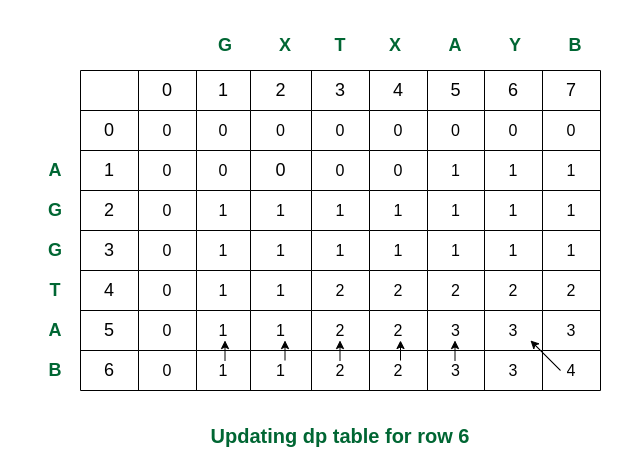Filling the final row

So we get the maximum length of common subsequence as 4.

Following is a tabulated implementation for the LCS problem.

## C++

 `// Dynamic Programming C implementation of LCS problem` `#include ` `using` `namespace` `std;`   `// Returns length of LCS for X[0..m-1], Y[0..n-1]` `int` `lcs(string X, string Y, ``int` `m, ``int` `n)` `{` `    ``// Initializing a matrix of size (m+1)*(n+1)` `    ``int` `L[m + 1][n + 1];`   `    ``// Following steps build L[m+1][n+1] in bottom up` `    ``// fashion. Note that L[i][j] contains length of LCS of` `    ``// X[0..i-1] and Y[0..j-1]` `    ``for` `(``int` `i = 0; i <= m; i++) {` `        ``for` `(``int` `j = 0; j <= n; j++) {` `            ``if` `(i == 0 || j == 0)` `                ``L[i][j] = 0;`   `            ``else` `if` `(X[i - 1] == Y[j - 1])` `                ``L[i][j] = L[i - 1][j - 1] + 1;`   `            ``else` `                ``L[i][j] = max(L[i - 1][j], L[i][j - 1]);` `        ``}` `    ``}`   `    ``// L[m][n] contains length of LCS for X[0..n-1] ` `    ``// and Y[0..m-1]` `    ``return` `L[m][n];` `}`   `// Driver code` `int` `main()` `{` `    ``string S1 = ``"AGGTAB"``;` `    ``string S2 = ``"GXTXAYB"``;` `    ``int` `m = S1.size();` `    ``int` `n = S2.size();`   `    ``cout << ``"Length of LCS is "` `<< lcs(S1, S2, m, n);`   `    ``return` `0;` `}` `// code submitted by Aditya Yadav (adityayadav012552)`

## Java

 `// Dynamic Programming Java implementation of LCS problem`   `import` `java.util.*;`   `public` `class` `LongestCommonSubsequence {`   `    ``// Returns length of LCS for X[0..m-1], Y[0..n-1]` `    ``int` `lcs(String X, String Y, ``int` `m, ``int` `n)` `    ``{` `        ``int` `L[][] = ``new` `int``[m + ``1``][n + ``1``];`   `        ``// Following steps build L[m+1][n+1] in bottom up` `        ``// fashion. Note that L[i][j] contains length of LCS` `        ``// of X[0..i-1] and Y[0..j-1]` `        ``for` `(``int` `i = ``0``; i <= m; i++) {` `            ``for` `(``int` `j = ``0``; j <= n; j++) {` `                ``if` `(i == ``0` `|| j == ``0``)` `                    ``L[i][j] = ``0``;` `                ``else` `if` `(X.charAt(i - ``1``) == Y.charAt(j - ``1``))` `                    ``L[i][j] = L[i - ``1``][j - ``1``] + ``1``;` `                ``else` `                    ``L[i][j] = max(L[i - ``1``][j], L[i][j - ``1``]);` `            ``}` `        ``}` `        ``return` `L[m][n];` `    ``}`   `    ``// Utility function to get max of 2 integers` `    ``int` `max(``int` `a, ``int` `b) { ``return` `(a > b) ? a : b; }`   `    ``public` `static` `void` `main(String[] args)` `    ``{` `        ``LongestCommonSubsequence lcs` `            ``= ``new` `LongestCommonSubsequence();` `        ``String S1 = ``"AGGTAB"``;` `        ``String S2 = ``"GXTXAYB"``;` `        ``int` `m = S1.length();` `        ``int` `n = S2.length();`   `        ``System.out.println(``"Length of LCS is"` `                           ``+ ``" "` `+ lcs.lcs(S1, S2, m, n));` `    ``}` `}`   `// This Code is Contributed by Saket Kumar`

## Python3

 `# Dynamic Programming implementation of LCS problem `   `def` `lcs(X , Y, m, n):      `   `    ``# Declaring the array for storing the dp values ` `    ``L ``=` `[[``None``]``*``(n``+``1``) ``for` `i ``in` `range``(m``+``1``)] `   `    ``# Following steps build L[m+1][n+1] in bottom up fashion ` `    ``# Note: L[i][j] contains length of LCS of X[0..i-1] ` `    ``# and Y[0..j-1]` `    ``for` `i ``in` `range``(m``+``1``): ` `        ``for` `j ``in` `range``(n``+``1``): ` `            ``if` `i ``=``=` `0` `or` `j ``=``=` `0` `: ` `                ``L[i][j] ``=` `0` `            ``elif` `X[i``-``1``] ``=``=` `Y[j``-``1``]: ` `                ``L[i][j] ``=` `L[i``-``1``][j``-``1``]``+``1` `            ``else``: ` `                ``L[i][j] ``=` `max``(L[i``-``1``][j] , L[i][j``-``1``]) `   `    ``# L[m][n] contains the length of LCS of X[0..n-1] & Y[0..m-1] ` `    ``return` `L[m][n] `     `# Driver code` `if` `__name__ ``=``=` `'__main__'``:` `    ``S1 ``=` `"AGGTAB"` `    ``S2 ``=` `"GXTXAYB"` `    ``m ``=` `len``(S1) ` `    ``n ``=` `len``(S2)` `    ``print` `(``"Length of LCS is"``, lcs(S1, S2, m, n) )`   `# This code is contributed by Nikhil Kumar Singh(nickzuck_007) `

## C#

 `// Dynamic Programming implementation of LCS problem` `using` `System;`   `class` `GFG {` `    ``// Returns length of LCS for X[0..m-1], Y[0..n-1]` `    ``static` `int` `lcs(String X, String Y, ``int` `m, ``int` `n)` `    ``{` `        ``int``[, ] L = ``new` `int``[m + 1, n + 1];`   `        ``// Following steps build L[m+1][n+1]` `        ``// in bottom up fashion.` `        ``// Note that L[i][j] contains length of` `        ``// LCS of X[0..i-1] and Y[0..j-1]` `        ``for` `(``int` `i = 0; i <= m; i++) {` `            ``for` `(``int` `j = 0; j <= n; j++) {` `                ``if` `(i == 0 || j == 0)` `                    ``L[i, j] = 0;` `                ``else` `if` `(X[i - 1] == Y[j - 1])` `                    ``L[i, j] = L[i - 1, j - 1] + 1;` `                ``else` `                    ``L[i, j] = max(L[i - 1, j], L[i, j - 1]);` `            ``}` `        ``}` `        ``return` `L[m, n];` `    ``}`   `    ``// Utility function to get max of 2 integers` `    ``static` `int` `max(``int` `a, ``int` `b) { ``return` `(a > b) ? a : b; }`   `    ``// Driver code` `    ``public` `static` `void` `Main()` `    ``{` `        ``String S1 = ``"AGGTAB"``;` `        ``String S2 = ``"GXTXAYB"``;` `        ``int` `m = S1.Length;` `        ``int` `n = S2.Length;`   `        ``Console.Write(``"Length of LCS is"` `                      ``+ ``" "` `+ lcs(S1, S2, m, n));` `    ``}` `}` `// This Code is Contributed by Sam007`

## PHP

 ` `

## Javascript

 ``

Output

`Length of LCS is  4`

Time Complexity: O(m * n) which is much better than the worst-case time complexity of Naive Recursive implementation.
Auxiliary Space: O(m * n) because the algorithm uses an array of size (m+1)*(n+1) to store the length of the common substrings.

### Usage of LCS Problem:

It is a classic computer science problem, the basis of diff (a file comparison program that outputs the differences between two files), and has applications in bioinformatics.

Related Articles: